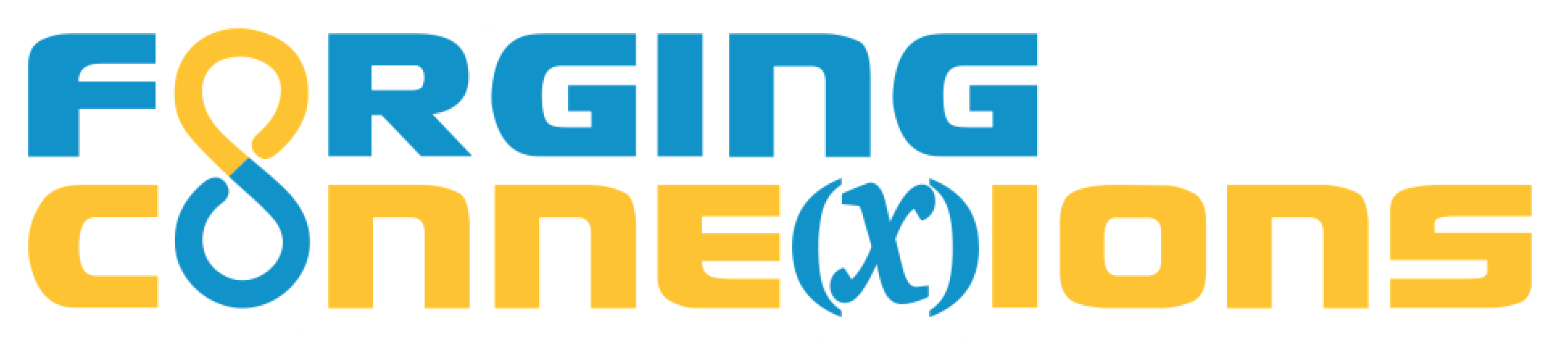Reconnecting Geometry and Algebra with Web Sketchpad

Introduction (5 min)

Scott Steketee, 21st Century Partnership for STEM Education, stek@21pstem.org

CCSSM expects students to understand transformations as functions. With Web Sketchpad, algebra students exploit this standard: Using geometric transformations they vary variables; experience domain, range, and rate of change; and connect their learning to the graph of y = mx + b.

Richard Feynman famously said “What I cannot create, I do not understand.” The focus of today’s session is to enable students to create their own functions with variables that students actually vary, with relative rate of change that they observe, analyze, and control, and with functions whose behavior creates patterns and pictures. These sensorimotor experiences of creating, manipulating, and observing mathematical objects are all aimed at helping students develop a deep and broad understanding of function concepts.

Agenda

1. Introduction (5 min)
2. Big Ideas (5 min)
3. Construct the Reflect Function (Participants, 15 min)
4. Restrict the Domain (5 min)
5. Introduce Dynagraphs (Pass the Mouse, 10 min)
6. Create a Cartesian Graph (25 min)
7. Discuss, Reflect, Q&A (Participants, 10 min)

Big Ideas (5 min)

As students construct, manipulate, and analyze transformations from a function point of view, they build conceptual understanding through a focus on the big ideas.

•

•

•

•

•

•

•

Construct the Reflect Function (15 min)

• Create an independent variable using the Point tool.
• Create a function rule using the Mirror tool.
• Create a dependent variable using the Reflect tool.

Drag x around. What do you notice about the relative speed and direction of x and r_j(x)?

• Turn on tracing for x and r_j(x) and draw an interesting shape. Include parts where you drag x toward and away from the mirror and parts where you drag it parallel to the mirror.

Draw a picture of your traces. Show the mirror in your drawing.

• You and your partner should each take a turn making a design of your own, and you should each submit a drawing of your traces to your teacher.

On page 1, the instructions said to use the Point tool first to create independent variable x, the Mirror tool to create mirror j, and the Reflect tool to create dependent variable r_j(x). The idea behind this was to emphasize the three parts of a function: the independent variable, the function rule, and the dependent variable.

The Reflect tool can actually create all three parts of a Reflect function, so you don't really need all three tools—but please be sure to keep in mind these three parts of any function that you work with.

Use the Reflect tool to construct a reflect function. Then adjust the function rule so that you can drag x to make the traces match the background image.

What other letters can you trace out by using a reflect function?

The fixed points of a function are the places where the independent variable x and the dependent variable r_j(x) come together.

Construct a reflect function and figure out where its fixed points are.

What do you notice about these fixed points? What do you wonder?

What do you think the symbols r_j(x) represent?

If you saw a dependent variable labeled r_p(z), what do you think this label would mean?

On this page, reflect point x across two different mirrors, j and k. Then trace to make an interesting design.

Is there more than one way to interpret this direction?

Make a drawing of your result.

On this page, see if you can figure out how to construct these dependent variables: r_j(r_k(x)) and r_k(r_j(x)).

Describe in words what these two labels mean.

How can you arrange j and k so that r_j(r_k(x)) and r_k(r_j(x)) lie on top of each other?

Make a drawing of your result.

Restrict the Domain (5 min)

What happens when students restrict the domain of the Dilate and Translate function families to a number line, and then compose these two functions?

Introduce Dynagraphs (10 min)

Create your own composed Dilate-and-Translate function restricted to a number line, modified to put the dependent variable on a second number line. Analyze how the dilation scale factor and translation vector affect the motion of the points, and how they relate the values of the variables. Finish by playing the Dynagraph game, trying to match the behavior of a mystery function.

Create a Cartesian Graph (25 min)

In the capstone of this series of activities, students create the Cartesian graph of a linear function using only dilation, translation, and rotation functions, and understand the shape of the resulting graph in terms of the dilation scale factor and the translation vector.

Discuss & Reflect (10 min)

How is this approach in keeping with Feynman’s observation about creating in order to understand?

How might it work in your own classroom?

What problems would you anticipate?

How might it encourage your students to notice, and to wonder?

What other questions and comments do you have?

Resources

▹︎︎ The Fine Print

Requirements:

These activities require web access using a browser that supports HTML5 and JavaScript. (That means almost any current browser.) No purchase is required, and there’s no advertising anywhere.

Release Information

[an error occurred while processing this directive]

Update History: